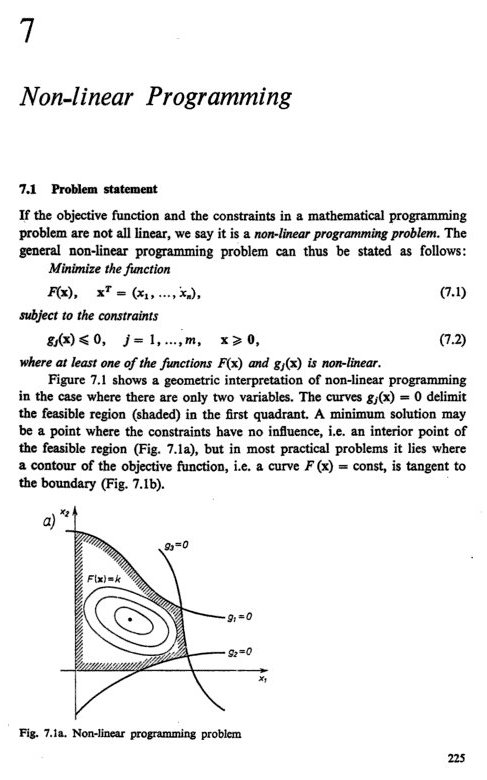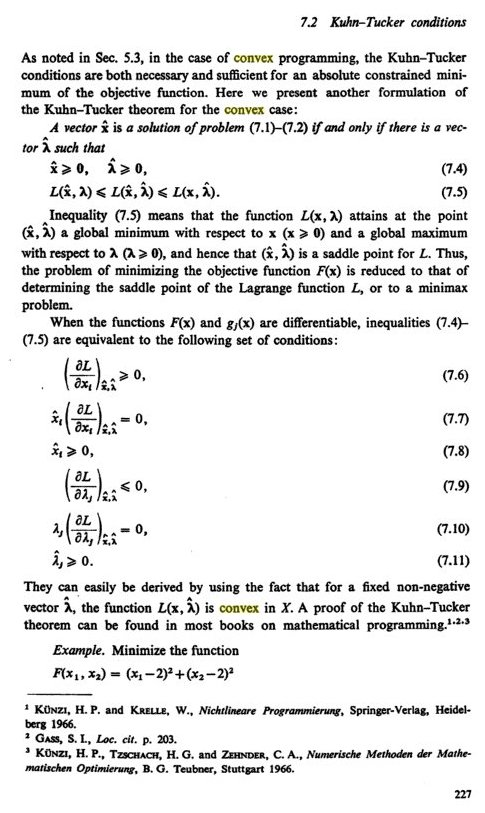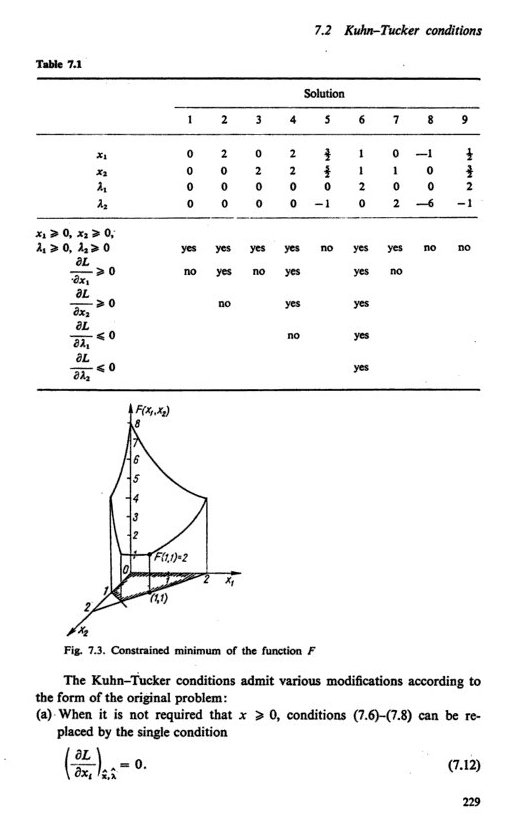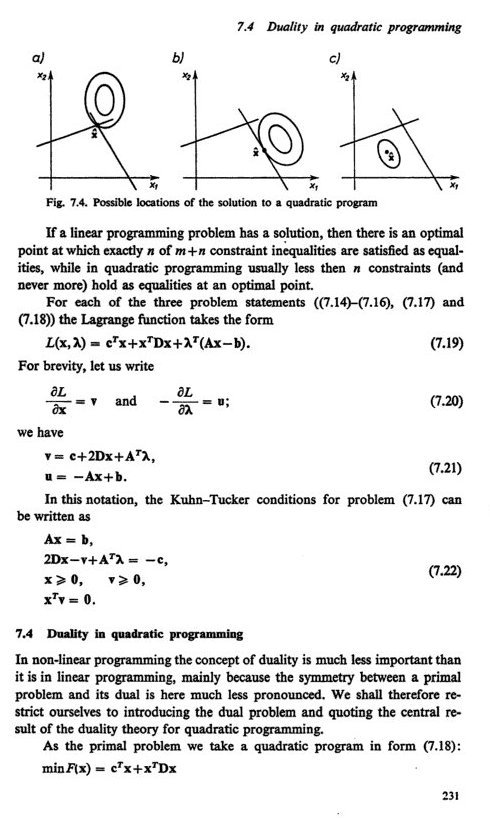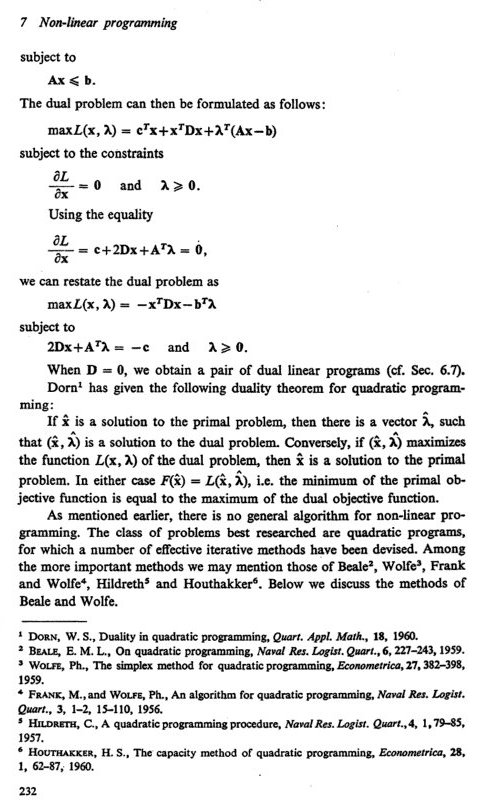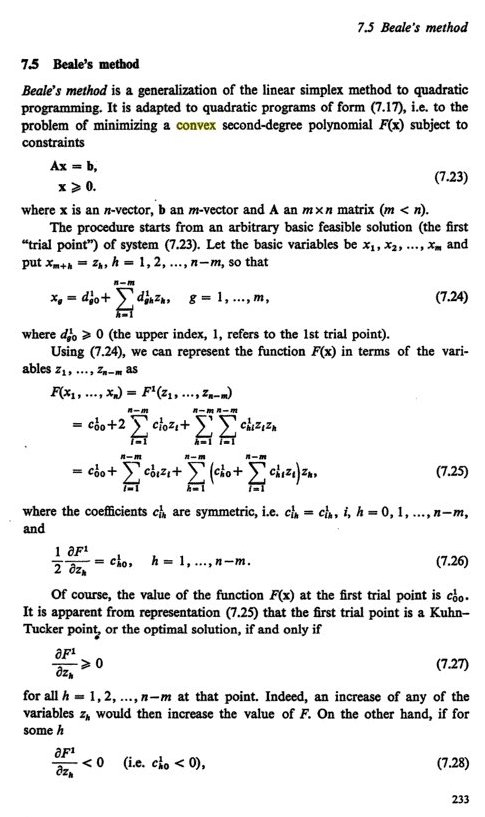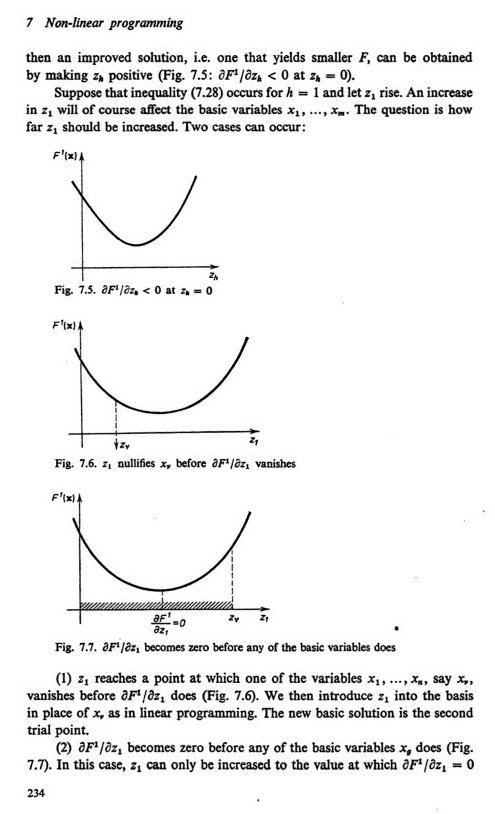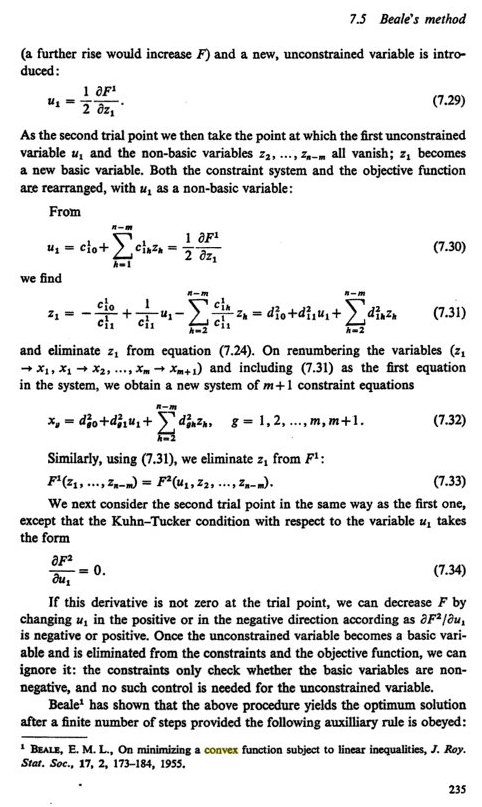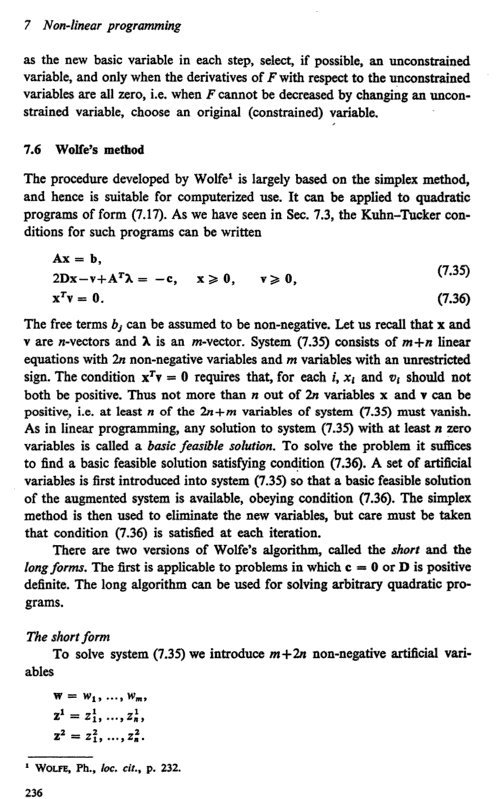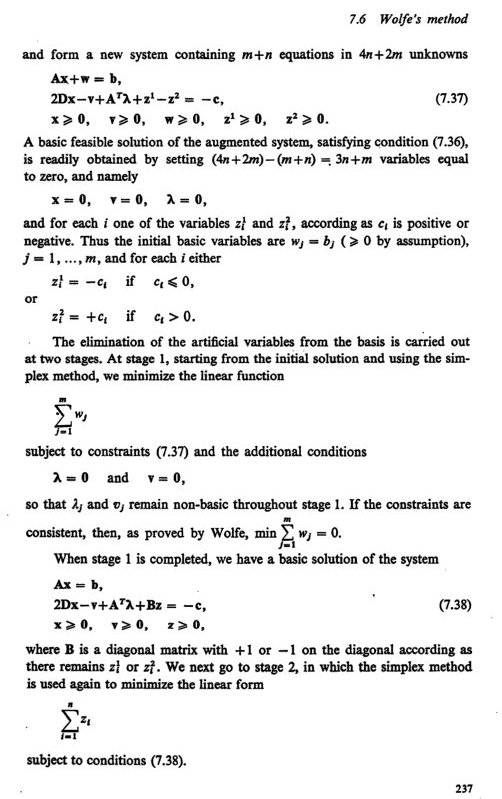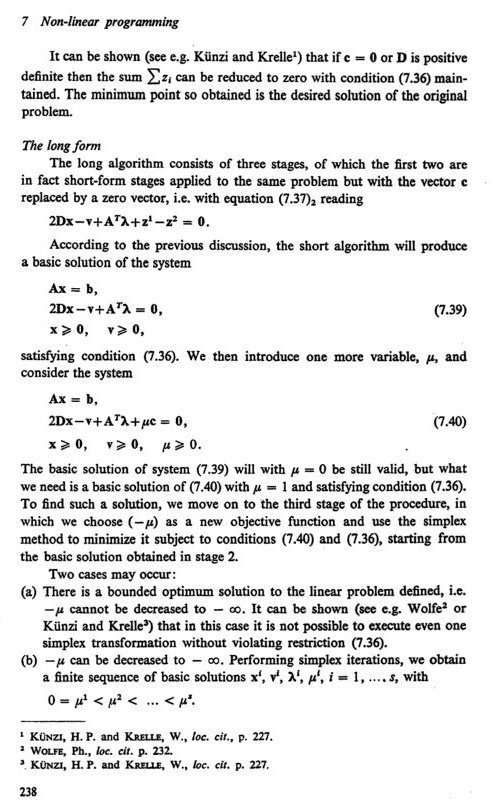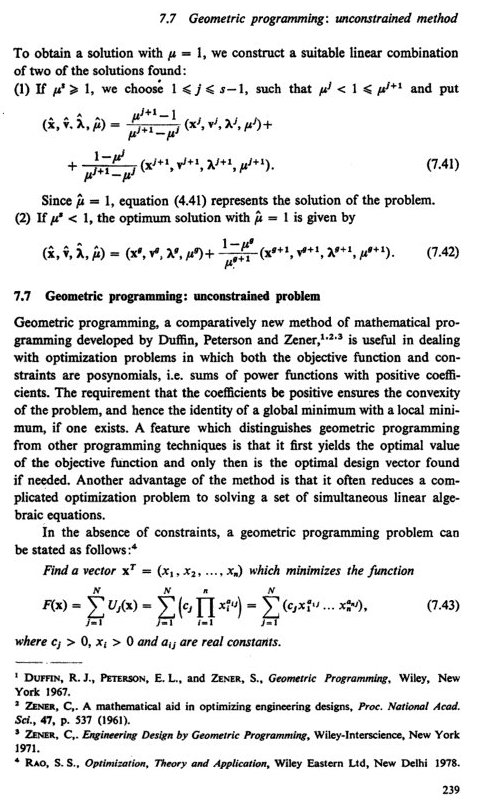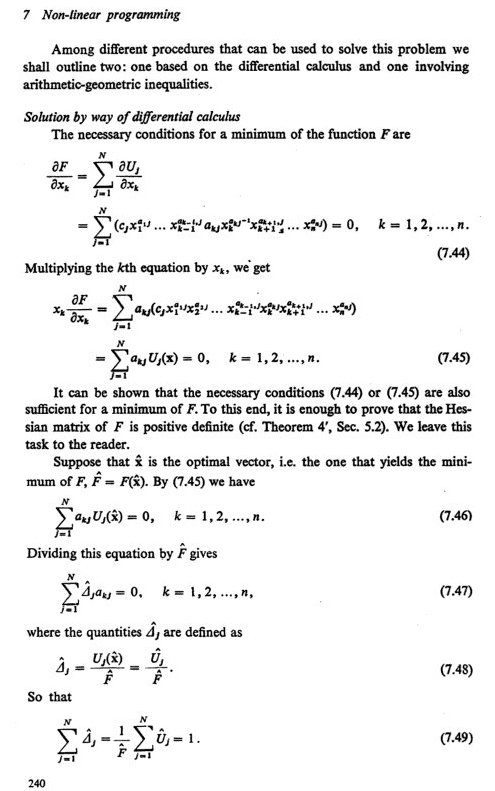Math 21a, Fall 2008
Exhibits page Math 21a 2008, Multivariable Calculus
Multivariable Calculus and Economics Glossary
Office: SciCtr 434Here is a very short glossary of some economic concepts and their explanations in mathematics (calculus). Thanks to the economist Jasminka Sohinger to suggest and explained to me (Oliver) some of the terms. (Any possible errors are of course mine):

Term Explanation
Cobb-Douglas function f(x,y) = A xalpha ybeta, example of an utility function. x,y are usually referred as goods or bundles of goods
Budget constraint special constraint g(x,y) = c, the constant c is the level of income
Utility function A function of two variables f(x,y) measuring the level of utility, the variables x,y typically represent goods. There can be more variables.
Production function A function of two variables f(x,y) measuring the level of production, x,y typically are labor and capital. There can be more variables.
Cost function A function of two variables f(x,y), where x,y are inputs The function can be a constraint. There can be more variables.
Objective function F Function of several variables f, to be maximized or minimized.
Program Problem
Duality Maximizing utility given expenditures or minimizing expenditures by fixing utility. Example in mathematics: minimize the surface area under fixed volume or maximize the volume when surface area is fixed.
Convex sets A set Y is convex if for all a in [0,1], and all x,y in Y also a x + (1-a) y is in Y. Economists prefer convexity since in decision processes, one wants to interpolate between two points x,y.
Convex function A function is convex on a convex set S if f(a*x + (1-a) y) smaller or equal than a*f(x) + (1-a)*f(y) for all a in [0,1] and all x,y in S. Example: f(x,y) = x2+y2 is convex. A function is concave on S, if -f is convex on S.
Quasiconvex function An utility function f(x,y) is quasiconvex if the lower level sets Yc = { f(x,y) smaller or equal than c } are convex sets. Example: f(x,y) = -xy is quasiconvex on the first quadrant S. Note that it is not convex because 1 = f( (1,1)) = f( 1/2( (0,0) + (2,2) ) ) is smaller than 1/2 [ f( (0,0)) + f (2,2)) ] = 2.
Quasiconcave function Utility function f(x,y) is quasiconcave if -f is quasiconcave. Equivalently, the upper level sets Yc = { f(x,y) larger or equal than c } are convex sets. Cobb-Douglas functions like f(x,y) = 3 x2 y3 are quasiconcave. (*)
Programming problem: Find the global minimum f on the region G defined by hi=0, gj less or equal to 0
Linear programming problem: Programming problem, where f and gi,hj are all linear.
Convex programming: The function f and the contraints gj are all convex
Quadratic programming: Contraints are linear, the objective function is a sum of a linear function and a quadratic form.
Kuhn-Tucker conditions Conditions for global minimum: convex f,gi, affine hj

 (*) What economists call quasiconcavity translates into ordinal (as opposed to cardinal) properties of the utility function. They will be preserved under positive monotone transformations of the utility function. This means that under positive monotone transformations of the utility function, even though its cardinal value will change, it will still represent the same preferences. A word about preferences: Economic theorists assume that a consumer has preferences that are described as binary relations. They call them "preference relations" and they have to satisfy certain properties for a utility function to be derived from them, such as completeness, transitivity, and continuity. Additional properties such as convexity of preferences, monotonicity, local insatiation, etc. are necessary for the theory to hold but are not indispensable for deriving the utility function. They all need convex level sets belonging to the utility function. The same logic applies when one analyzes production using the Cobb Douglas function as a production function describing technology. There, however, we don't start with preferences. Production analysis is simpler because it is observable and measurable. Consumer analysis is more complex (and fun).

## Some pages of "Criteria and Methods of Structural Optimization" by Andrzej Marek Brandt